2019-11-08 16:00:35 qq_38190041 阅读数 69
• ###### MATLAB图像处理

全面系统的学习MATLAB在图像处理中的应用

19905 人正在学习 去看看 魏伟

### 1.任务

PCB图的直线提取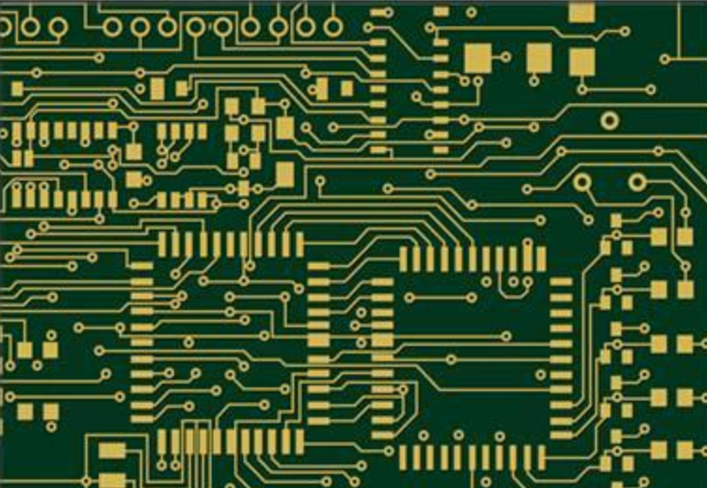Windows10专业版
VS2015企业版
C++ opencv3.2

### 3.图像处理的思路

Hough变换直线检测

##### 直线检测-Hough_Line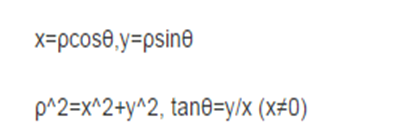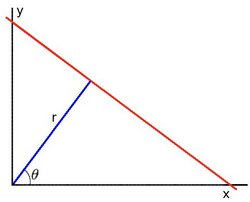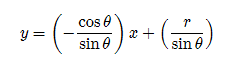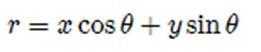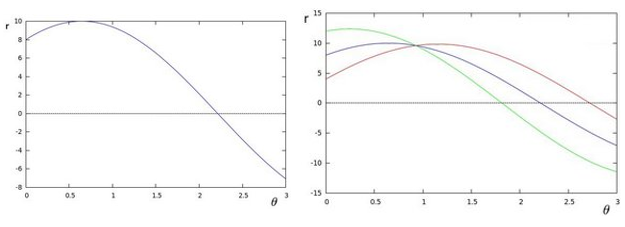``````cv::HoughLinesP(
InputArray src, // 输入图像，必须8-bit的灰度图像
OutputArray lines, // 输出的极坐标来表示直线
double rho, // 生成极坐标时候的像素扫描步长
double theta, //生成极坐标时候的角度步长，一般取值CV_PI/180
int threshold, // 阈值，只有获得足够交点的极坐标点才被看成是直线
double minLineLength=0;// 最小直线长度
double maxLineGap=0;// 最大间隔
)

``````

#### 第一种：灰度二值化后，不做其他处理

1.读入图像
2.将图像转为灰度
3.将灰度图像二值化
4.Hough直线检测
5.将直线用红线标记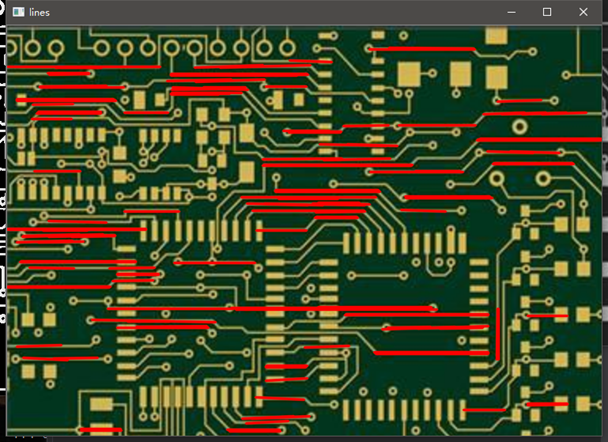1.读入图像
2.将图像转为灰度
3.将灰度图像二值化
4.使用较大结构元素腐蚀后膨胀剩下大块区域
5.使用二值化图像减去大块区域剩下直线等小像素
6.Hough直线检测
7.将直线用红线标记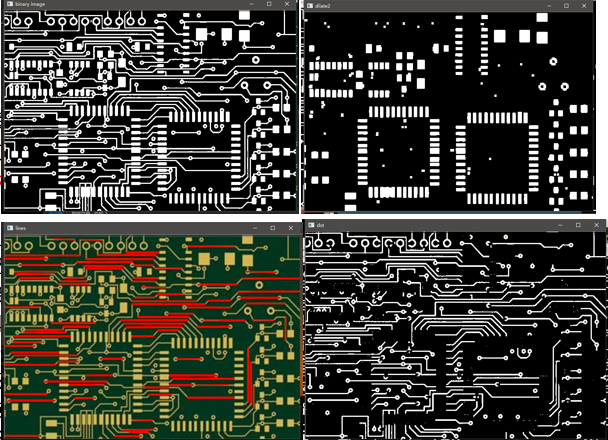1.读入图像
2.将图像转为灰度
3.将灰度图像二值化
4.使用长水平矩形结构元素做开操作(腐蚀+膨胀)提取水平直线
5.使用长垂直矩形结构元素做开操作(腐蚀+膨胀)提取垂直直线
6.两提取图像相加
6.Hough直线检测
7.将直线用红线标记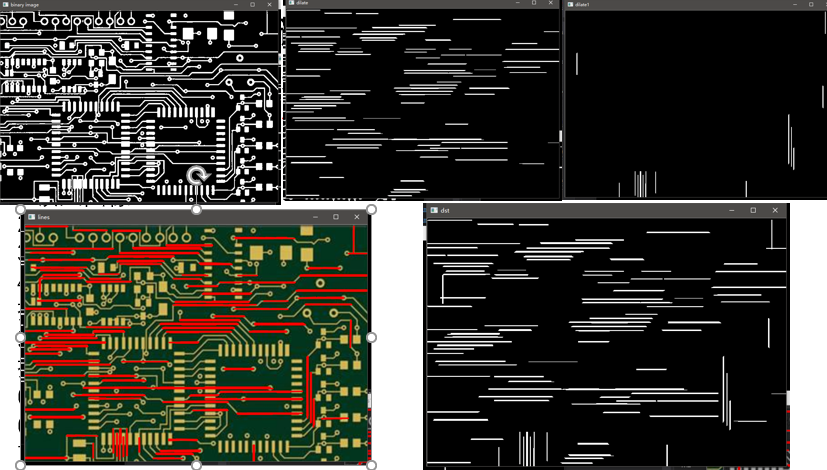### 总结

1.图像处理的方法有很多种，不是唯一，单一的。
2.图像的预处理是比较关键的，这直接影响着最后的检测结果的好坏。

``````#include <iostream>
#include <opencv2\opencv.hpp>
#include <stdio.h>
using namespace cv;
using namespace std;

Mat src, gray, dst, binaryImage, morhpImage1 , morhpImage2, kernel;
int main(int argc, char **argv)
{
imshow("src image", src);

cvtColor(src, gray, CV_BGR2GRAY);

// 二值化
threshold(gray, binaryImage, 0, 255, THRESH_BINARY | THRESH_OTSU);
imshow("binary image", binaryImage);

int height = 60;
int width = 60;

// 可以直接使用形态学开闭操作的函数
kernel = getStructuringElement(MORPH_RECT, Size(width, 1), Point(-1, -1));
erode(binaryImage, morhpImage1, kernel);
imshow("erode", morhpImage1);

kernel = getStructuringElement(MORPH_RECT, Size(width, 1), Point(-1, -1));
dilate(morhpImage1, morhpImage1, kernel);
imshow("dilate", morhpImage1);

kernel = getStructuringElement(MORPH_RECT, Size(1, height), Point(-1, -1));
erode(binaryImage, morhpImage2, kernel);
imshow("erode1", morhpImage2);

kernel = getStructuringElement(MORPH_RECT, Size(1, height), Point(-1, -1));
dilate(morhpImage2, morhpImage2, kernel);
imshow("dilate1", morhpImage2);

dst = morhpImage1 + morhpImage2;
imshow("dst", dst);

vector<Vec4i>lines;
HoughLinesP(dst, lines, 1, CV_PI / 180.0, 30, 20.0, 0);
Mat resultImage = src.clone();
Mat retImage = gray.clone();
cvtColor(retImage, retImage, COLOR_GRAY2BGR);
for (size_t t = 0; t < lines.size(); t++)
{
Vec4i ln = lines[t];
line(resultImage, Point(ln, ln), Point(ln, ln), Scalar(0, 0, 255), 2, LINE_AA, 0);
line(retImage, Point(ln, ln), Point(ln, ln), Scalar(0, 0, 255), 2, LINE_AA, 0);
}
imshow("lines", resultImage);
imshow("lines1", retImage);
waitKey(0);

return 0;
}
``````

2017-07-26 13:35:29 walilk 阅读数 11180
• ###### MATLAB图像处理

全面系统的学习MATLAB在图像处理中的应用

19905 人正在学习 去看看 魏伟

### 前言

[图像处理] 实验笔记系列是以图像处理算法为主的文章专栏，以我在算法研究中的实验笔记资料为基础加以整理推出的。该系列内容涉及常见的图像处理算法理论以及常见的算法应用，每篇博客都会介绍相关的算法原理，代码实现和算法在实际应用中的技巧。
本文主要整理自笔者在一项图像处理任务中的直线检测（line detection）部分的笔记资料，采用了基于霍夫变换（Hough Transform）的直线检测算法。文中给出了直线检测常用的算法介绍，论文资料等，以及笔者的实验笔记和实验结果。

文章小节安排如下：
1）直线检测相关算法
2）霍夫直线检测的基本原理
3）霍夫直线检测的OpenCV实现
4）直线检测的应用

### 一、直线检测相关算法

#### 1.1 霍夫变换（Hough Transform）

霍夫变换（Hough Transform）换于1962年由Paul Hough 首次提出，后于1972年由Richard Duda和Peter Hart推广使用，是图像处理中从图像中检测几何形状的基本方法之一。经典霍夫变换用来检测图像中的直线，后来霍夫变换经过扩展可以进行任意形状物体的识别，例如圆和椭圆。

霍夫变换运用两个坐标空间之间的变换将在一个空间中具有相同形状的曲线或直线映射到另一个坐标空间的一个点上形成峰值，从而把检测任意形状的问题转化为统计峰值问题。

参考论文：
 P.V.C. Hough,Machine Analysis of Bubble Chamber Pictures, Proc. Int. Conf. High Energy Accelerators and Instrumentation, 1959.
 Duda, R. O. and P. E. Hart, “Use of the Hough Transformation to Detect Lines and Curves in Pictures,”Comm. ACM, Vol. 15, pp. 11–15 (January, 1972).

#### 1.2 霍夫直线检测（Hough Line Detection）

Hough直线检测的基本原理在于利用点与线的对偶性，在我们的直线检测任务中，即图像空间中的直线与参数空间中的点是一一对应的，参数空间中的直线与图像空间中的点也是一一对应的。这意味着我们可以得出两个非常有用的结论：
1）图像空间中的每条直线在参数空间中都对应着单独一个点来表示；
2）图像空间中的直线上任何一部分线段在参数空间对应的是同一个点。

因此Hough直线检测算法就是把在图像空间中的直线检测问题转换到参数空间中对点的检测问题，通过在参数空间里寻找峰值来完成直线检测任务。

-待续-

### 二、霍夫直线检测的基本原理

#### 2.1 关于对偶性

首先，我们通过实例来解释一下对偶性的意义。
1）图像空间中的点与参数空间中的直线一一对应
在图像空间x-y中一条直线在直角坐标系下可以表示为：其中k和b是参数，对应表示斜率和截距。过某一点A(x0, y0)的所有直线的参数均满足方程y0=k*x0+b，即点A(x0, y0)确定了一族直线。
如果我们将方程改写为：那么该方程在参数空间k-b中就对应了一条直线：也就是说，图像空间x-y中的点(x0,y0)对应了参数空间k-b中的直线b=-k*x0+y0。因此可以得到结论，图像空间中的点与参数空间中的直线一一对应。

2）图像空间中的直线与参数空间中的点一一对应
我们在直线y=k*x+b上再增加一个点B(x1, y1)，如下图所示：那么点B(x1, y1)在参数空间同样对应了一条直线：可以看到，图像空间x-y中的点A和点B在参数空间k-b中对应的直线相交于一点，这也就是说AB所确定的直线，在参数空间中对应着唯一一个点，这个点的坐标值（k0, b0）也就是直线AB的参数。

以上就是在直线检测任务中关于对偶性的直观解释。这个性质也为我们解决直线检测任务提供了方法，也就是把图像空间中的直线对应到参数空间中的点，最后通过统计特性来解决问题。假如图像空间中有两条直线，那么最终在参数空间中就会对应到两个峰值点，依此类推。

#### 2.2 参数空间的选择

上述为了方便讲解对偶性和霍夫变换的基本原理，我们的参数空间也选择了笛卡尔直角坐标系。但在实际应用中，参数空间是不能选择直角坐标系的，因为原始图像直角坐标空间中的特殊直线x=c（垂直x轴，直线的斜率为无穷大）是没办法在基于直角坐标系的参数空间中表示的。

所以在实际应用中，参数空间采用极坐标系ρ-θ，图示如下：直线的表达式为：化简便可得到：对于直线上的点(x0, y0)，可以将通过该点的直线族定义为：这就回到我们刚才的结论，参数空间的每个点(ρ,θ)都对应了图像空间的一条直线，或者说图像空间的一个点在参数空间中就对应为一条曲线。参数空间采用极坐标系，这样就可以在参数空间表示原始空间中的所有直线了。
注意，此时图像空间（直角坐标系x-y）上的一个点对应到参数空间（极坐标系ρ-θ）上是一条曲线，确切的说是一条正弦曲线。#### 2.3 利用霍夫变换检测直线

如前所述，霍夫直线检测就是把图像空间中的直线变换到参数空间中的点，通过统计特性来解决检测问题。具体来说，如果一幅图像中的像素构成一条直线，那么这些像素坐标值（x, y）在参数空间对应的曲线一定相交于一个点，所以我们只需要将图像中的所有像素点（坐标值）变换成参数空间的曲线，并在参数空间检测曲线交点就可以确定直线了。

在理论上，一个点对应无数条直线或者说任意方向的直线，但在实际应用中，我们必须限定直线的数量（即有限数量的方向）才能够进行计算。

因此，我们将直线的方向θ离散化为有限个等间距的离散值，参数ρ也就对应离散化为有限个值，于是参数空间不再是连续的，而是被离散量化为一个个等大小网格单元。将图像空间（直角坐标系）中每个像素点坐标值变换到参数空间（极坐标系）后，所得值会落在某个网格内，使该网格单元的累加计数器加1。当图像空间中所有的像素都经过霍夫变换后，对网格单元进行检查，累加计数值最大的网格，其坐标值（ρ0, θ0）就对应图像空间中所求的直线。以上就是霍夫直线检测算法要做的，它检测图像中每个像素点在参数空间对应曲线之间的交点，如果交于一点的曲线的数量超过了阈值，那就可以认为这个交点(ρ,θ)在图像空间中对应一条直线。

#### 2.4 霍夫直线检测的优缺点

优点：
Hough直线检测的优点是抗干扰能力强，对图像中直线的殘缺部分、噪声以及其它共存的非直线结构不敏感。
缺点：
Hough变换算法的特点导致其时间复杂度和空间复杂度都很高，并且在检测过程中只能确定直线方向，丢失了线段的长度信息。

### 三、霍夫直线检测的OpenCV实现

OpenCV支持三种霍夫直线检测算法：
1）Standard Hough Transform(SHT，标准霍夫变换)
2）Multiscale Hough Transform(MSHT，多尺度霍夫变换)
3）Progressive Probability Houth Transform(PPHT，渐进概率式霍夫变换)

#### 3.1 霍夫直线检测函数定义

在OpenCV2.1之前的版本，霍夫直线检测函数如下：

函数原型：

CVAPI(CvSeq*) cvHoughLines2( CvArr* image, void* line_storage, int method,
double rho, double theta, int threshold,
double param1 CV_DEFAULT(0), double param2 CV_DEFAULT(0),
double min_theta CV_DEFAULT(0), double max_theta CV_DEFAULT(CV_PI));

函数说明：
cvHoughLines2老版OpenCV的霍夫直线检测函数，通过method参数可以支持三种霍夫直线检测算法，分别是CV_HOUGH_STANDARD、CV_HOUGH_PROBABILISTIC =1、CV_HOUGH_MULTI_SCALE。

在OpenCV新版本下，霍夫直线检测算法定义了两个函数：HoughLines、HoughLinesP
1）HoughLines：标准霍夫变换、多尺度霍夫变换
函数原型：

CV_EXPORTS_W void HoughLines( InputArray image, OutputArray lines,
double rho, double theta, int threshold,
double srn = 0, double stn = 0,
double min_theta = 0, double max_theta = CV_PI );

参数说明：
InputArray image：输入图像，必须是8位单通道图像。
OutputArray lines：检测到的线条参数集合。
double rho：以像素为单位的距离步长。
double theta：以弧度为单位的角度步长。
int threshold：累加计数值的阈值参数，当参数空间某个交点的累加计数的值超过该阈值，则认为该交点对应了图像空间的一条直线。
double srn：默认值为0，用于在多尺度霍夫变换中作为参数rho的除数，rho=rho/srn。
double stn：默认值为0，用于在多尺度霍夫变换中作为参数theta的除数，theta=theta/stn。

函数说明：
HoughLines函数输出检测到直线的矢量表示集合，每一条直线由具有两个元素的矢量（ρ, θ）表示，其中ρ表示直线距离原点（0, 0）的长度，θ表示直线的角度（以弧度为单位）。
HoughLines函数无法输出图像空间中线段的长度，这也是霍夫变换本身的弱点。

备注说明：
如果srn和stn同时为0，就表示HoughLines函数执行标准霍夫变换，否则就是执行多尺度霍夫变换。

2）HoughLinesP：渐进概率式霍夫变换
函数原型：

CV_EXPORTS_W void HoughLinesP( InputArray image, OutputArray lines,
double rho, double theta, int threshold,
double minLineLength = 0, double maxLineGap = 0 );

参数说明：
InputArray image：输入图像，必须是8位单通道图像。
OutputArray lines：检测到的线条参数集合。
double rho：直线搜索时的距离步长，以像素为单位。
double theta：直线搜索时的角度步长，以弧度为单位。
int threshold：累加计数值的阈值参数，当参数空间某个交点的累加计数的值超过该阈值，则认为该交点对应了图像空间的一条直线。
double minLineLength：默认值为0，表示最小线段长度阈值（像素）。
double maxLineGap：默认值为0，表示直线断裂的最大间隔距离阈值。即如果有两条线段是在一条直线上，但它们之间有间隙，那么如果这个间隔距离大于该值，则被认为是一条线段，否则认为是两条线段。

函数说明：
HoughLinesP函数输出检测到直线的矢量表示集合，每一条直线由具有四个元素的矢量（x1, y1, x2, y2）表示，其中(x1, y1)表示线段的起点，(x2, y2)表示线段的终点。
HoughLinesP函数可以检测出图像空间中线段的长度。

#### 3.2 霍夫直线检测函数使用

霍夫直线变换是一种用来在图像空间寻找直线的方法，输入图像要求是二值图像，同时为了提高检测直线的效率和准确率，在使用霍夫线变换之前，最好对图像进行边缘检测生成边缘二值图像，这样的检测效果是最好的。

1）HoughLines函数
代码：

``````std::string img_path;
cv::Mat mat_color;
cv::Mat mat_gray;
cv::Mat mat_binary;
cv::Mat mat_canny;
cv::Mat mat_board;

img_path = "line.png";
mat_board = cv::Mat(mat_color.size(), mat_color.type(), Scalar::all(255));

// binary
cv::threshold(mat_gray, mat_binary, 0.0, 255.0, cv::THRESH_OTSU);
// invert color
cv::bitwise_not(mat_binary, mat_binary);

// detect edge
Canny(mat_binary, mat_canny, 50, 200, 3);

// detect line
vector<Vec2f> lines;
HoughLines(mat_canny, lines, 1, CV_PI / 180, 150, 0, 0);

// draw line
cout << "line number: " << lines.size() << endl;
for (size_t i = 0; i < lines.size(); i++)
{
Vec2f linex = lines[i];
cout << "radius: " << linex << ", radian: "<< linex << ", angle: " << 180 / CV_PI * linex << endl;
float rho = lines[i], theta = lines[i];
Point pt1, pt2;
double a = cos(theta), b = sin(theta);
double x0 = a * rho, y0 = b * rho;
pt1.x = cvRound(x0 + 1000 * (-b));
pt1.y = cvRound(y0 + 1000 * (a));
pt2.x = cvRound(x0 - 1000 * (-b));
pt2.y = cvRound(y0 - 1000 * (a));
line(mat_board, pt1, pt2, Scalar(255, 0, 0), 1);
}
cv::imshow("gray", mat_gray);
cv::imshow("binary", mat_binary);
cv::imshow("canny", mat_canny);
cv::imshow("color", mat_board);
cv::waitKey();``````

原图：二值图：边缘图：检测效果：2）HoughLinesP函数
代码：

``````std::string img_path;
cv::Mat mat_color;
cv::Mat mat_gray;
cv::Mat mat_binary;
cv::Mat mat_canny;
cv::Mat mat_board;

img_path = "line.png";
mat_board = cv::Mat(mat_color.size(), mat_color.type(), Scalar::all(255));

// binary
cv::threshold(mat_gray, mat_binary, 0.0, 255.0, cv::THRESH_OTSU);
// invert color
cv::bitwise_not(mat_binary, mat_binary);

// detect edge
Canny(mat_binary, mat_canny, 50, 200, 3);

// detect line
vector<Vec4i> lines;
HoughLinesP(mat_canny, lines, 1, CV_PI / 180, 150, 50, 50);

// draw line
cout << "line number: " << lines.size() << endl;
for (size_t i = 0; i < lines.size(); i++)
{
Vec4i linex = lines[i];
line(mat_board, Point(linex, linex), Point(linex, linex), Scalar(255, 0, 0), 1);
}

cv::imshow("gray",   mat_gray);
cv::imshow("binary", mat_binary);
cv::imshow("canny", mat_canny);
cv::imshow("color", mat_board);
cv::waitKey();``````

检测效果：### 四、直线检测的应用

#### 4.1 直线检测的实际应用

直线检测是机器视觉和模式识别中最重要的任务之一，对图像理解/分析等有重要的意义。在实际应用中，直线检测可用于机器人定位中的网格识别，板材的裂纹检测，表单票据的格式识别，零件纹路的检测，自动驾驶中的车道检测等等，可以看出，在工业领域中，直线检测以及各种图像处理技术应用是非常丰富的。

#### 4.2 直线检测在图像矫正方面的应用

笔者最近在一个OCR项目也使用了Hough Line Detection算法。在该项目中，待识别文本图像的内容是倾斜的（即文字是倾斜的），我们采用的测略就是通过直线检测确定图像内容的倾斜程度并进行旋转纠正，这样得到的无倾斜图像更有利于OCR任务。
原图：矫正效果：### 五、参考资料

 Duda, R. O. and P. E. Hart, “Use of the Hough Transformation to Detect Lines and Curves in Pictures,”Comm. ACM, Vol. 15, pp. 11–15 (January, 1972).
 顾思妍. 机器视觉的直线检测技术及应用研究[D].广东工业大学,2011.
 数字图像处理[M]. 电子工业出版社 , (美)RafaelC.Gonzalez,(美)RichardE.Woods,(美)StevenL.Eddins著, 2005

Hough变换-理解篇
http://blog.csdn.net/abcjennifer/article/details/7448513
Hough transform(霍夫变换)
http://www.cnblogs.com/AndyJee/p/3805594.html

http://www.jianshu.com/p/55eabb42c6c2

2019-04-10 20:11:24 matt45m 阅读数 605
• ###### MATLAB图像处理

全面系统的学习MATLAB在图像处理中的应用

19905 人正在学习 去看看 魏伟

## 前言

1.直线检测在好多实现应用中能用到到，我之前做过扫描件检测时用直线检测来处理判断页面是否水平，还有在辅助驾驶中的车道偏离预警系统也有过应用。
2.我的编程环境是Windows 7 64位，IDE是VS2015，配置了OpenCV3.3与OpenCV_Contrib,实现语言是C++。是于如果配置以上的环境，可以看我之前写的博文。

## 一、概述

1.直线检测的原理介绍
（1）对于直角坐标系中的任意一点A(x0,y0)，经过点A的直线满足Y0=kX0+b.(k是斜率，b是截距)。
（2）那么在X-Y平面过点A(x0,y0)的直线簇可以用Y0=k
X0+b表示，但对于垂直于X轴的直线斜率是无穷大的则无法表示。因此将直角坐标系转换到极坐标系就能解决该特殊情况。
（3）在极坐标系中表示直线的方程为ρ=xCosθ+ySinθ（ρ为原点到直线的距离）。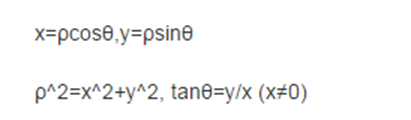（4）对于任意一条直线上的所有点来说，变换到极坐标中，从[0~360]空间，可以得到r的大小，属于同一条直线上点在极坐标空(r, theta)必然在一个点上有最强的信号出现，根据此反算到平面坐标中就可以得到直线上各点的像素坐标。从而得到直线。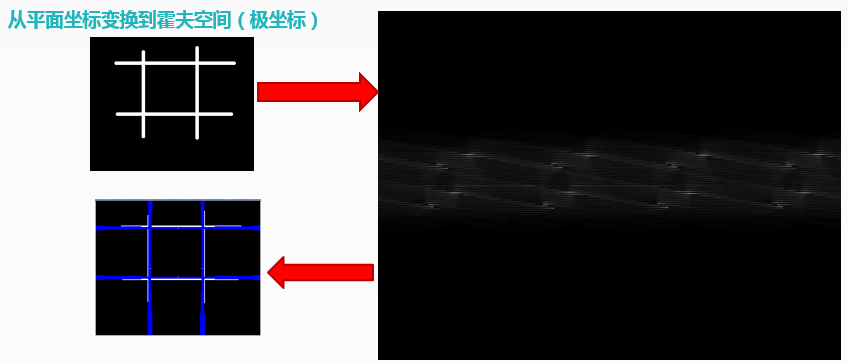2.OpenCV封装有直线检测的函数，HoughLines（）和HoughLinesP（），它们都能实现直线检测，差别是：HoughLines（）函数使用标准的Hough变换HoughLinesP（）函数使用概率Hough变换，即只通过分析点的子集并估计这些点都属于一条直线的概率，这在计算速度上更快。
（1）HoughLines（）函数说明：
HoughLines(
InputArray src, // 输入图像，必须8-bit的灰度图像
OutputArray lines, // 输出的极坐标来表示直线
double rho, // 生成极坐标时候的像素扫描步长
double theta, //生成极坐标时候的角度步长，一般取值CV_PI/180
int threshold, // 阈值，只有获得足够交点的极坐标点才被看成是直线
double srn = 0;// 是否应用多尺度的霍夫变换，如果不是设置0表示经典霍夫变换
double stn = 0;//是否应用多尺度的霍夫变换，如果不是设置0表示经典霍夫变换
double min_theta = 0; // 表示角度扫描范围 0 ~180之间， 默认即可
double max_theta = CV_PI
)
（2）HoughLinesP（）函数说明：
HoughLinesP(
InputArray src, // 输入图像，必须8-bit的灰度图像
OutputArray lines, // 输出的极坐标来表示直线
double rho, // 生成极坐标时候的像素扫描步长
double theta, //生成极坐标时候的角度步长，一般取值CV_PI/180
int threshold, // 阈值，只有获得足够交点的极坐标点才被看成是直线
double minLineLength = 0;// 最小直线长度
double maxLineGap = 0;// 最大间隔
)

## 二、代码演示

1.传入一张图像，把所有直线画出来。

``````void lineDetection(string image_path)
{
Mat src, src_gray, dst;
if (!src.data)
{
std::cerr << "无法打开图像文件！" << endl;
return;
}

namedWindow("input image", CV_WINDOW_AUTOSIZE);
namedWindow("hough-line-detection", CV_WINDOW_AUTOSIZE);
imshow("input image", src);

//边缘检测
Canny(src, src_gray, 150, 200);
//灰度化
cvtColor(src_gray, dst, CV_GRAY2BGR);

vector<Vec2f> lines;
//霍夫曼直线检测
Point pt1, pt2;
HoughLines(src_gray, lines, 1, CV_PI / 180, 150, 0, 0);
for (size_t i = 0; i < lines.size(); i++)
{
// 极坐标中的r长度
float rho = lines[i];
// 极坐标中的角度
float theta = lines[i];
double a = cos(theta), b = sin(theta);
double x0 = a*rho, y0 = b*rho;
// 转换为平面坐标的四个点
pt1.x = cvRound(x0 + 1000 * (-b));
pt1.y = cvRound(y0 + 1000 * (a));
pt2.x = cvRound(x0 - 1000 * (-b));
pt2.y = cvRound(y0 - 1000 * (a));
line(dst, pt1, pt2, Scalar(0, 0, 255), 1, CV_AA);
}

imshow("matt", src);

imshow("hough-line-detection", dst);

waitKey(0);
}
``````

2.运行结果## 结语

1.例子很简单，没有什么难度，但应用时肯定要按自己的数据去做相关参数的设定。
2.关于工程的源码，运行程序时的bug,都可以加这个群(487350510)互相讨论学习。

2011-11-18 20:54:01 liujia2100 阅读数 13454
• ###### MATLAB图像处理

全面系统的学习MATLAB在图像处理中的应用

19905 人正在学习 去看看 魏伟

Hough变换：

for(i=0;i<img.height;i++) //转化成灰度

{

for(j=0,n=0;n<img.width*3,j<img.width;n+=3,j++)

{ //gray 变量存储rgb转灰度的数据

gray= ((float)(img.image[lineBytes*i+n+2])+(float)(*(img.image+lineBytes*i+n+1))+(float)(*(img.image+lineBytes*i+n)))/3; //lineBytes 原图每行实际字节数

grayPic[i*img.width+j]=(byte)gray;//转换成的灰度图像放在grayPic中

}

}

int logNum; //边缘检测

memset(lpDIBBits,(byte)0,sizeof(byte)*img.height*img.width);

for(i=3;i<img.height-2;i++)

for(j=3;j<img.width-2;j++)

{

//logNum 变量 记录每次运算的值

logNum=16*grayPic[i*img.width+j]-grayPic[(i-2)*img.width+j]-grayPic[(i-1)*img.width+j-1]-2*grayPic[(i-1)*img.width+j]-grayPic[(i-1)*img.width+j+1]-grayPic[i*img.width+j-2]-2*grayPic[i*img.width+j-1]-2*grayPic[i*img.width+j+1]-grayPic[i*img.width+j+2]-grayPic[(i+1)*img.width+j-1]-2*grayPic[(i+1)*img.width+j]-grayPic[(i+1)*img.width+j+1]-grayPic[(i+2)*img.width+j];//log算子

if(logNum > 0)

lpDIBBits[i*img.width+j]=255;//边缘检测后的数据存放在lpDIBBits中

else

lpDIBBits[i*img.width+j]=0;

}

for(i=1;i<img.height;i++) //img.height原图高度

for(j=1;j<img.width;j++) //img.width 原图宽度

{

if(lpDIBBits[i*img.width+j]==255) //对边缘检测后的数据（存在lpDIBBits中）进行hough变化

{

for(k=1;k<ma;k++) //ma=180

{

p=(int)(i*cos(pi*k/180)+j*sin(pi*k/180));//p hough变换中距离参数

p=(int)(p/2+mp/2); //p值优化防止为负

npp[k][p]=npp[k][p]++; //npp对变换域中对应重复出现的点累加

}

}

}

kmax=0; //最长直线的角度

pmax=0; //最长直线的距离

n=0; //这一部分为寻找最长直线

for(i=1;i<ma;i++) //ma=180

for(j=1;j<mp;j++) //mp为原图对角线距离

{

if(npp[i][j]>yuzhi) //找出最长直线 yuzhi为中间变量用于比较

{

yuzhi=npp[i][j];

kmax=i; //记录最长直线的角度

pmax=j; //记录最长直线的距离

}

}

memset(temp,(byte)255,sizeof(byte)*img.width*img.height);//原图中坐标符合kmax和pmax的值

for(i=1;i<img.height;i++) //的集合即是最长的直线

for(j=1;j<img.width;j++)

{

if(lpDIBBits[i*img.width+j]==255)

{

p=(int)(i*cos(pi*kmax/180)+j*sin(pi*kmax/180));//pi=3.1415926

p=(int)(p/2+mp/2); //mp为原图对角线距离

if(p==pmax)

*(temp+i*img.width+j)=0; //存储图像数据 放在temp数组中

}

}2017-06-20 15:45:35 u011574296 阅读数 623
• ###### MATLAB图像处理

全面系统的学习MATLAB在图像处理中的应用

19905 人正在学习 去看看 魏伟